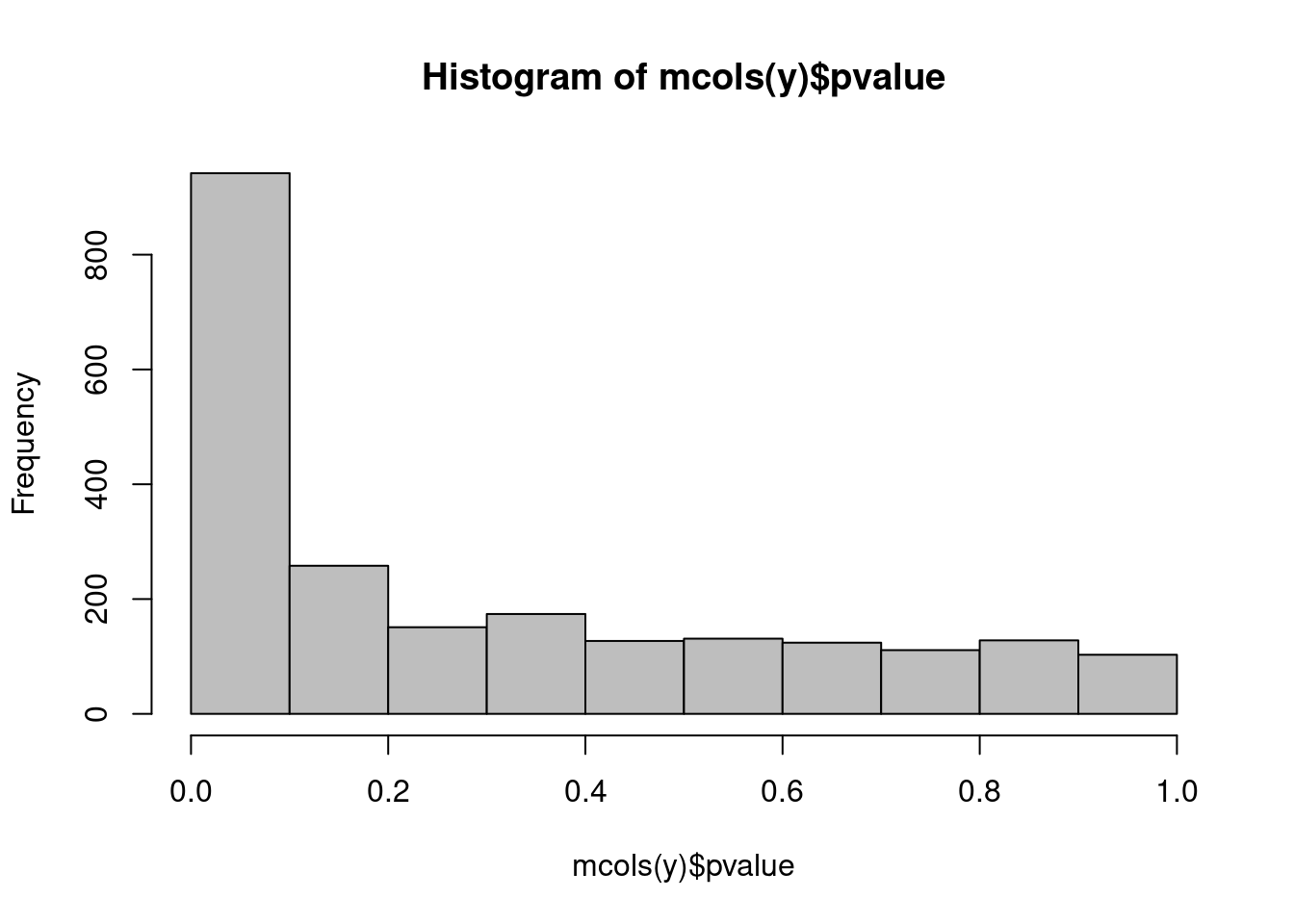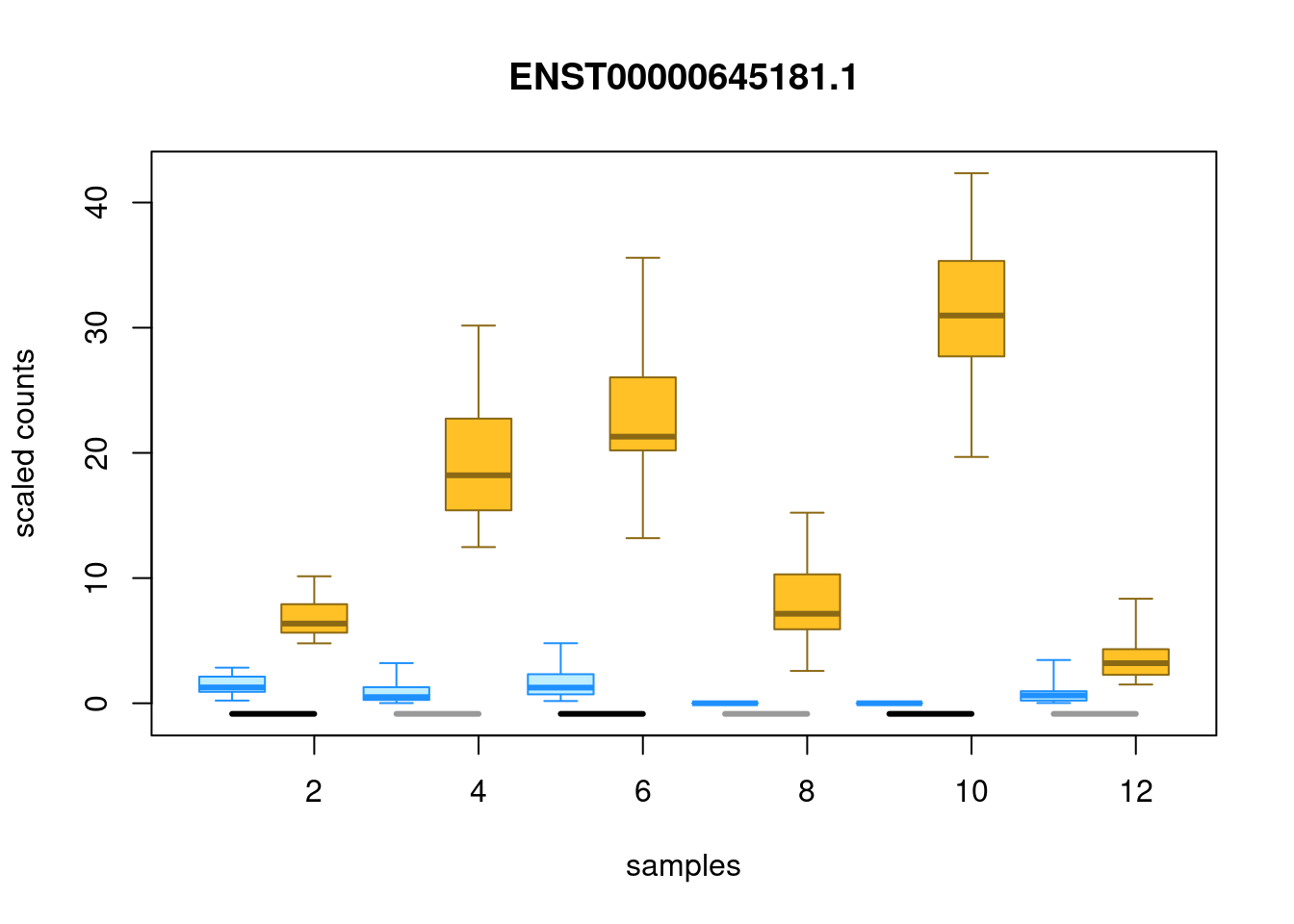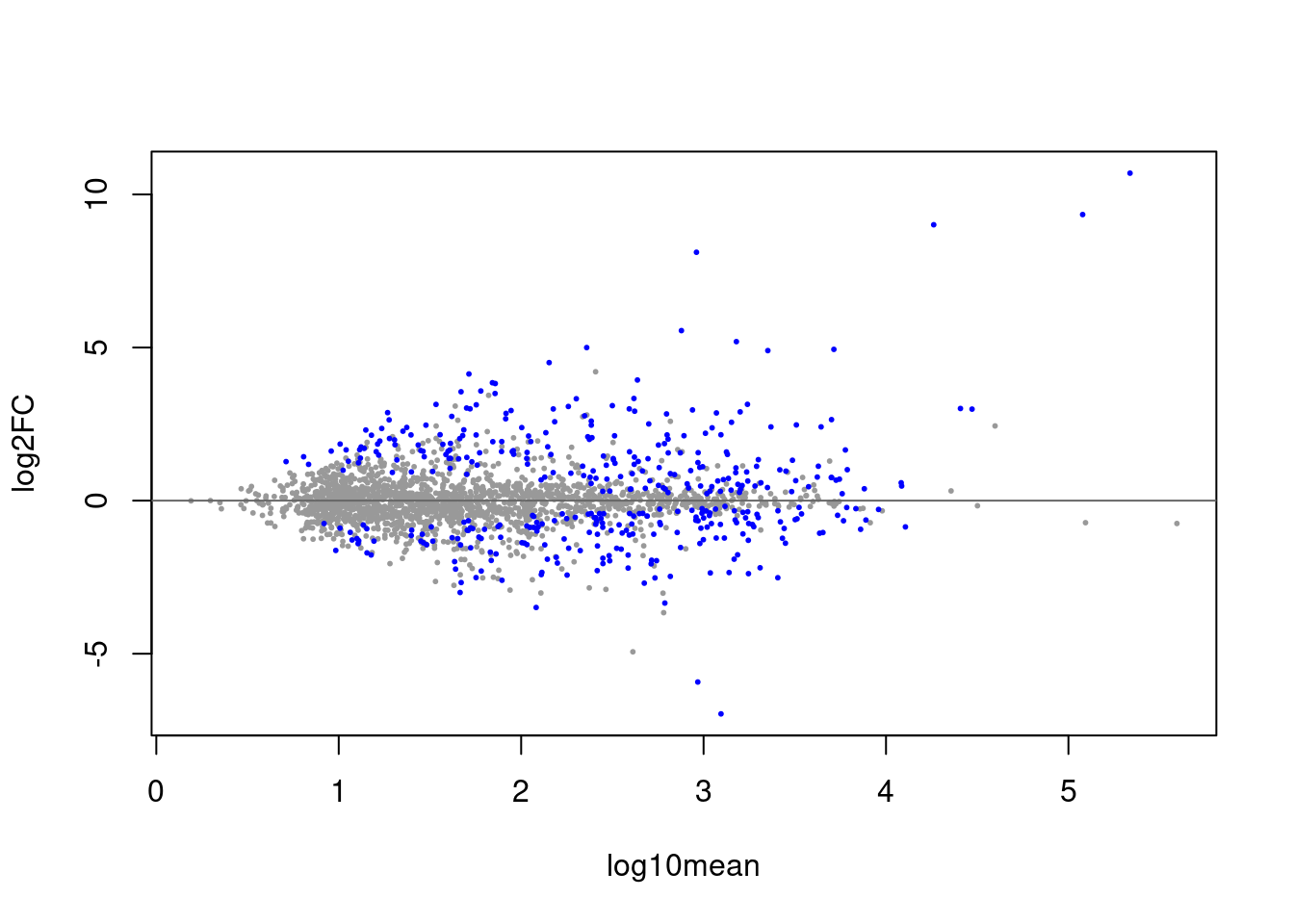# The Swish method

The Swish method for differential expression analysis of bulk or single-cell RNA-seq data using inferential replicate counts is described in the following reference: Zhu et al. (2019) doi: 10.1093/nar/gkz622.

We note that Swish extends and builds on another method, SAMseq (Li and Tibshirani 2011), implemented in the samr package, by taking into account inferential uncertainty, and allowing to control for batch effects and matched samples. Additionally, Swish has methods for testing changes in effect size across secondary covariates, which we refer to as “interactions”. Swish also implements correlation tests as in the original SAMseq package. `swish` calls functions from the qvalue (Storey and Tibshirani 2003) or samr package for calculation of local FDR and q-value. This vignette gives an example of differential analysis of matched samples, and an interaction test for matched samples, to see if a condition effect changes in magnitude across two groups of samples.

# Quick start

The following lines of code will perform a basic transcript-level `swish` two group analysis of bulk RNA-seq. For more details, read on. There is a special section below for two-group analysis of scRNA-seq.

``````# 'coldata.csv': sample information table
library(tximeta)
y <- tximeta(coldata) # reads in counts and inf reps
library(fishpond)
y <- scaleInfReps(y) # scales counts
y <- labelKeep(y) # labels features to keep
set.seed(1)
y <- swish(y, x="condition") # simplest Swish case``````

Note: Inferential replicates, either from Gibbs sampling or bootstrapping of reads, are required for the swish method. When running Salmon, you can set `--numGibbsSamples 30` to generate Gibbs samples, or `--numBootstraps 30` to generate bootstrap samples (we typically recommend 20-30 inferential replicates).

The results can be found in `mcols(y)`. For example, one can calculate the number of genes passing a 5% FDR threshold:

``table(mcols(y)\$qvalue < .05)``

One can at any point remove the genes that didn’t pass the expression filter with the following line of code (can be run before or after `swish`). These genes are ignored by `swish`, and so will have `NA` in the results columns in `mcols(y)`.

``y <- y[mcols(y)\$keep,]``

A gene-level analysis looks identical to a transcript-level analysis, only the input data changes. Examples follow.

Lastly, what is the structure of the output of `tximeta` (Love et al. 2020), which is used in `swish`? See the section below, Structure of tximeta output / swish input.

## Macrophage stimulation experiment

We begin the fishpond vignette by loading data from a Bioconductor Experiment Data package, macrophage. The package contains RNA-seq quantification from 24 RNA-seq samples, which are a subset of the RNA-seq samples generated and analyzed by Alasoo et al. (2018) - doi: 10.1038/s41588-018-0046-7.

The experiment involved treatment of macrophage cell lines from a number of human donors with IFN gamma, Salmonella infection, or both treatments combined. In the beginning of this vignette, we will focus on comparing the IFN gamma stimulated cell lines with the control cell lines, accounting for the paired nature of the data (cells from the same donor). Later in the vignette we will analyze differences in the Salmonella infection response by IFN gamma treatment status – whether the cells are primed for immune response.

We load the package, and point to the `extdata` directory. For a typical analysis, the user would just point `dir` to the location on the machine or cluster where the transcript quantifications are stored (e.g. the `quant.sf` files).

``````library(macrophage)
dir <- system.file("extdata", package="macrophage")``````

The data was quantified using Salmon (Patro et al. 2017) 0.12.0 against the Gencode v29 human reference transcripts (Frankish, GENCODE-consoritum, and Flicek 2018). For more details and all code used for quantification, refer to the macrophage package vignette.

Importantly, `--numGibbsSamples 20` was used to generate 20 inferential replicates with Salmon’s Gibbs sampling procedure. We also recommend to use `--gcBias` when running Salmon to protect against common sample-specific biases present in RNA-seq data.

# Data import

## Read in the column data from CSV

We start by reading in a CSV with the column data, that is, information about the samples, which are represented as columns of the SummarizedExperiment object we will construct containing the counts of reads per gene or transcript.

``````coldata <- read.csv(file.path(dir, "coldata.csv"))
``````##            names sample_id line_id replicate condition_name macrophage_harvest
## 1 SAMEA103885102    diku_A  diku_1         1          naive          11/6/2015
## 2 SAMEA103885347    diku_B  diku_1         1           IFNg          11/6/2015
## 3 SAMEA103885043    diku_C  diku_1         1         SL1344          11/6/2015
## 4 SAMEA103885392    diku_D  diku_1         1    IFNg_SL1344          11/6/2015
## 5 SAMEA103885182    eiwy_A  eiwy_1         1          naive         11/25/2015
## 6 SAMEA103885136    eiwy_B  eiwy_1         1           IFNg         11/25/2015
##   salmonella_date ng_ul_mean rna_extraction rna_submit        library_pool
## 1      11/13/2015   293.9625     11/30/2015  12/9/2015 3226_RNAauto-091215
## 2      11/13/2015   293.9625     11/30/2015  12/9/2015 3226_RNAauto-091215
## 3      11/13/2015   293.9625     11/30/2015  12/9/2015 3226_RNAauto-091215
## 4      11/13/2015   293.9625     11/30/2015  12/9/2015 3226_RNAauto-091215
## 5       12/2/2015   193.5450      12/3/2015  12/9/2015 3226_RNAauto-091215
## 6       12/2/2015   193.5450      12/3/2015  12/9/2015 3226_RNAauto-091215
##   chemistry rna_auto
## 1   V4_auto        1
## 2   V4_auto        1
## 3   V4_auto        1
## 4   V4_auto        1
## 5   V4_auto        1
## 6   V4_auto        1``````

We will subset to certain columns of interest, and re-name them for later.

``````coldata <- coldata[,c(1,2,3,5)]
names(coldata) <- c("names","id","line","condition")``````

`coldata` needs to have a column `files` which specifies the path to the quantification files. In this case, we’ve gzipped the quantification files, so we point to the `quant.sf.gz` file. We make sure that all the files exist in the location we specified.

``````coldata\$files <- file.path(dir, "quants", coldata\$names, "quant.sf.gz")
all(file.exists(coldata\$files))``````
``##  TRUE``

## Read in quants with tximeta

We will read in quantification data for some of the samples. First we load the SummarizedExperiment package. We will store out data and the output of the statistical method in a SummarizedExperiment object. We use the tximeta (Love et al. 2020) package to read in the data:

``suppressPackageStartupMessages(library(SummarizedExperiment))``

The macrophage package contains a number of samples under different conditions. In this vignette, we will demonstrate a two group comparison, and so we first subset our `coldata` to the `"naive"` and `"IFNg"` groups. Log fold changes will be made comparing `IFNg` to `naive` (the reference level).

``````coldata <- coldata[coldata\$condition %in% c("naive","IFNg"),]
coldata\$condition <- factor(coldata\$condition,
levels=c("naive","IFNg"))``````

We load in the quantification data with `tximeta`:

``````library(tximeta)
se <- tximeta(coldata)``````

We can see that all the assays have been loaded:

``assayNames(se)``
``````##   "counts"    "abundance" "length"    "infRep1"   "infRep2"   "infRep3"
##   "infRep4"   "infRep5"   "infRep6"   "infRep7"   "infRep8"   "infRep9"
##  "infRep10"  "infRep11"  "infRep12"  "infRep13"  "infRep14"  "infRep15"
##  "infRep16"  "infRep17"  "infRep18"  "infRep19"  "infRep20"``````

`tximeta` loads transcript-level data, although it can later be summarized to the gene levels:

``head(rownames(se))``
``````##  "ENST00000456328.2" "ENST00000450305.2" "ENST00000488147.1"
##  "ENST00000619216.1" "ENST00000473358.1" "ENST00000469289.1"``````

We will rename our SummarizedExperiment `y` for the statistical analysis. For speed of the vignette, we subset to the transcripts on chromosome 4.

``````y <- se
y <- y[seqnames(y) == "chr4",]``````

Note on factor levels: The `swish` function compares expression level across factors such that log2 fold changes are reported as the non-reference level over the reference level. By default, R will choose a reference level for factors based on alphabetical order, unless `levels` are explicitly set. It is recommended to set the factors levels, as in the above code chunk, with the reference level coming first in the character vector, so that log2 fold changes correspond to the comparison of interest.

# Differential transcript expression

## Running Swish at the transcript level

Running `swish` has three steps: scaling the inferential replicates, labeling the rows with sufficient counts for running differential expression, and then calculating the statistics. As `swish` makes use of pseudo-random number generation in breaking ties and in calculating permutations, to obtain identical results, one needs to set a random seed before running `swish()`, as we do below.

``````library(fishpond)
y <- scaleInfReps(y)
y <- labelKeep(y)
y <- y[mcols(y)\$keep,]
set.seed(1)
y <- swish(y, x="condition", pair="line")``````

Note: the simple paired test is the slowest of all the other designs provided for Swish (see further details), because it requires recomputing the signed ranks at each permutation. Other designs avoid recomputing ranks per permutation, or don’t use ranks for the test statistic. You can set `fast=1` for simple paired designs, which implements a one-sample z-score as the test statistic, and permutations to provide p-values and q-values. These permutations are much faster than those that use the signed rank test, for example with one core, the signed rank test is 12x slower than the one-sample z-score method when run across all transcripts, and they nevertheless detect nearly the same sets.

``````y_fast <- swish(y, x="condition", pair="line", fast=1)
table(original=mcols(y)\$qvalue < .1,
fast=mcols(y_fast)\$qvalue < .1)``````
``````##         fast
## original FALSE TRUE
##    FALSE  1615   40
##    TRUE     41  553``````

The default number of permutations for computing p-values is `nperms=100`. However, for paired datasets as this one, you may have fewer maximum permutations. In this case, there are 64 possible ways to switch the condition labels for six pairs of samples. We can set the `nperms` manually to 64, or if we had just used the default value, `swish` would set `nperms` to the maximum value possible and notify the user that it had done so.

A note about `labelKeep`: by default we keep features with `minN=3` samples with a minimal count of 10. For scRNA-seq data with de-duplicated UMI counts, we recommend to lower the count, e.g. a count of 3, across a higher number of `minN` cells, depending on the number of cells being compared. You can also set `x="condition"` when running `labelKeep` which will use the condition variable to set `minN`.

The results are stored in `mcols(y)`. We will show below how to pull out the top up- and down-regulated transcripts.

We can see how many transcripts are in a 5% FDR set:

``table(mcols(y)\$qvalue < .05)``
``````##
## FALSE  TRUE
##  1845   404``````

A number of features may have the same `pvalue` and `qvalue` due to the procedure for assessing significance. We can additionally rank features by their effect size, to break ties in the `qvalue`:

``````most.sig <- with(mcols(y),
order(qvalue, -abs(log2FC)))
``````## DataFrame with 6 rows and 2 columns
##                      log2FC     qvalue
##                   <numeric>  <numeric>
## ENST00000264888.5  10.69374 7.2338e-05
## ENST00000306602.2   9.34219 7.2338e-05
## ENST00000306621.7   9.00874 7.2338e-05
## ENST00000435136.6   8.11050 7.2338e-05
## ENST00000307128.5  -6.96429 7.2338e-05
## ENST00000382114.8  -5.92382 7.2338e-05``````

The top 6 genes by `qvalue` and effect size in this case all have positive LFC, although we have ranked by the largest in absolute value, so large negative values will also appear in this list.

## Plotting results

We can check the distribution of p-values. This looks as expected for a comparison where we expect many transcripts will be affected by the treatment (IFNg stimulation of macrophage cells). There is a flat component and then an enrichment of transcripts with p-values near 0.

``hist(mcols(y)\$pvalue, col="grey")``Of the transcripts in this set, which have the most extreme log2 fold change? Note that often many transcripts will share the same q-value, so it’s valuable to look at the log2 fold change as well (see further note below on q-value computation). The log2 fold change computed by `swish` is the median over inferential replicates, and uses a pseudo-count of 5 on the scaled counts, to stabilize the variance on the fold change from division by small counts. See the note above for interpretation of log2 fold changes with respect to the levels of `x`.

With the following code chunk, we construct two vectors that give the significant genes with the lowest (most negative) and highest (most positive) log2 fold changes.

``````with(mcols(y),
table(sig=qvalue < .05, sign.lfc=sign(log2FC))
)``````
``````##        sign.lfc
## sig      -1   1
##   FALSE 998 847
##   TRUE  184 220``````
``````sig <- mcols(y)\$qvalue < .05
lo <- order(mcols(y)\$log2FC * sig)
hi <- order(-mcols(y)\$log2FC * sig)``````

Here we print a small table with just the calculated statistics for the large positive log fold change transcripts (up-regulation):

``````top_up <- mcols(y)[head(hi),]
names(top_up)``````
``````##   "tx_id"     "gene_id"   "tx_name"   "log10mean" "keep"      "stat"
##   "log2FC"    "pvalue"    "locfdr"    "qvalue"``````
``````cols <- c("log10mean","log2FC","pvalue","qvalue")
print(as.data.frame(top_up)[,cols], digits=3)``````
``````##                   log10mean log2FC   pvalue   qvalue
## ENST00000264888.5      5.34  10.69 6.95e-06 7.23e-05
## ENST00000306602.2      5.08   9.34 6.95e-06 7.23e-05
## ENST00000306621.7      4.26   9.01 6.95e-06 7.23e-05
## ENST00000435136.6      2.96   8.11 6.95e-06 7.23e-05
## ENST00000502843.5      2.88   5.55 6.95e-06 7.23e-05
## ENST00000500655.2      3.18   5.19 6.95e-06 7.23e-05``````

Likewise for the largest negative log fold change transcripts (down-regulation):

``````top_down <- mcols(y)[head(lo),]
print(as.data.frame(top_down)[,cols], digits=3)``````
``````##                   log10mean log2FC   pvalue   qvalue
## ENST00000307128.5      3.10  -6.96 6.95e-06 7.23e-05
## ENST00000382114.8      2.97  -5.92 6.95e-06 7.23e-05
## ENST00000381753.8      2.08  -3.49 4.04e-03 3.34e-02
## ENST00000237612.7      2.79  -3.35 6.95e-06 7.23e-05
## ENST00000407365.5      1.67  -3.00 5.70e-03 3.86e-02
## ENST00000395002.6      2.67  -2.70 6.95e-06 7.23e-05``````

We can plot the scaled counts for the inferential replicates, and also group the samples by a covariate, in this case the cell line. The analysis was paired, so the statistic assessed if the change within pairs was consistent. Here we plot the 100th top up-regulated transcript. `plotInfReps` also has functionality for plotting uncertainty in single cell data, as will be covered in a later section.

``plotInfReps(y, idx=hi, x="condition", cov="line")``We can make an MA plot, where the transcripts in our FDR set are colored:

``plotMASwish(y, alpha=.05)``Using the `addIds` function from tximeta, we can easily add gene symbols. By specifying `gene=TRUE`, this will use the gene ID to match to gene symbols for all of the transcripts.

``````library(org.Hs.eg.db)
``````plotMASwish(y, alpha=.05, xlim=c(.5,5.5))